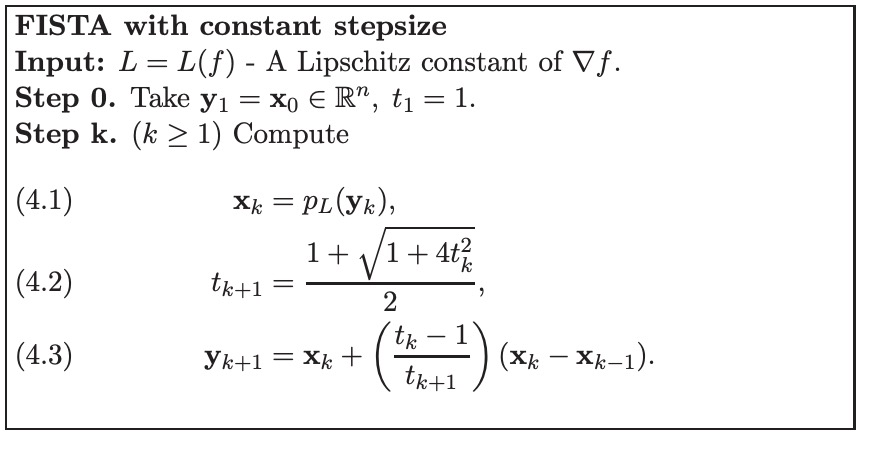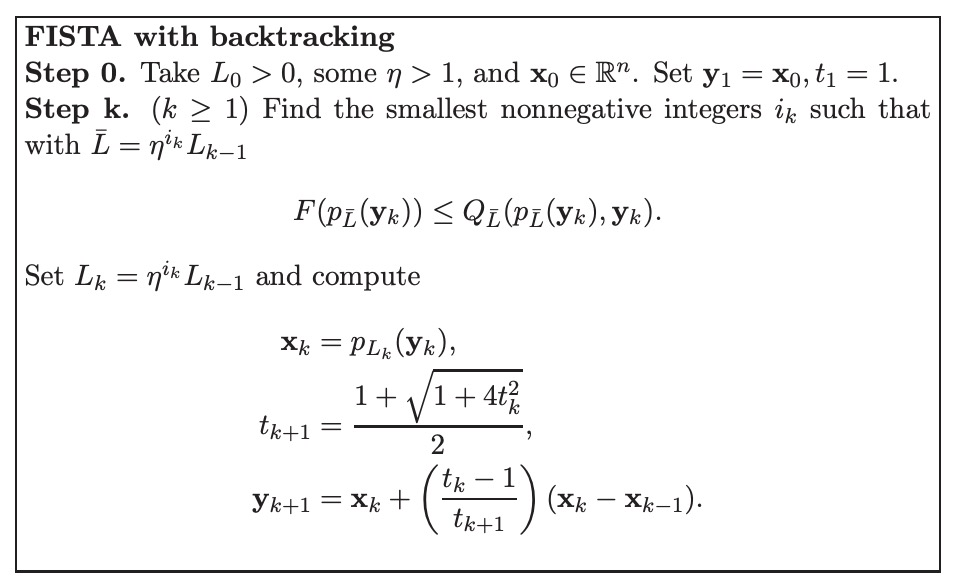Fater Iterative Shrinking Threshold Algorithm

Posted by GwanSiu on October 27, 2018

1. Introduction: Proximal Mapping

The definition of proximal mapping of convex function $h$ is:

2. Faster Iterative Shrinking Threshold Algorithm

The basic idea of the iterative shrinkage algorithm is to build at each iteration a regularization of the linearized differentiable function part in the objective. We consider the following general formualtion:

$g:\mathcal{R}^{n}\rightarrow\mathcal{R}$ is a continuous convex function but is possibly non-smooth. $f:\mathcal{R}^{n}\rightarrow\mathcal{R}$ is a smooth convex function such that Lipschitz continuous gradient $L(f)$:

where $\Arrowvert \cdot\Arrowvert$ denotes the euclidean distance and $L(f)$ is the Lipschitz constant of $\nabla f(x)$.

For any $L>0$, we use quadratic upper bound to approximate $F(x)=f(x)+g(x)$ at a given point $y$:

thus, we can use proximal gradient method to compute the minimum value of $Q(x,y)$:

In bothe ISTA and FISTA, update point $x_{k}$ is computed by proximal gradient method:

Faster iterative shrink threshold alorithm is shown as below: